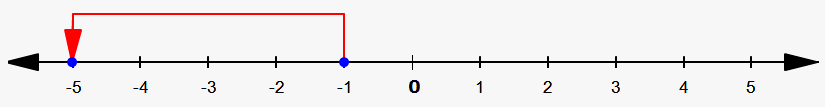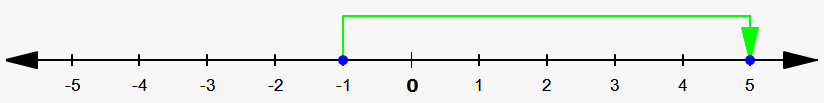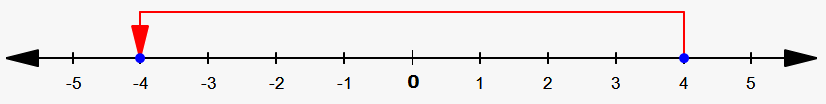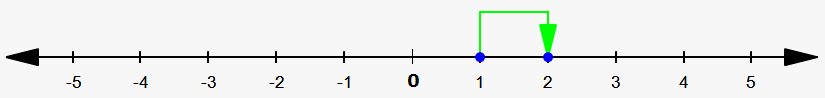Subtracting Integers on a Number Line

With the help of number line we can subtract integers.

It is better to show on examples how to do this.

Example 1. Find -1-4.

We draw -1 on a number line. Since we subtract positive number (4) we move 4 units to the left. Result is -5. So, -1-4=-5.Let's see how to subtract negative numbers.

Example 2. Find -1-(-6).

We draw -1 on a number line. Since we subtract negative number (-6) we move 6 units to the right. Result is 5. So, -1-(-6)=5.Now, do the following exercise to remember this topic better.

Exercise 1. Find 4-8.